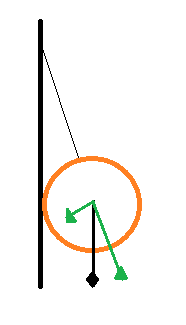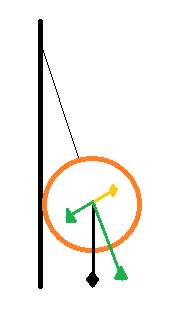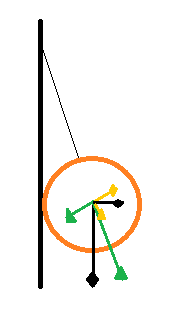# Where does the force come from?

• I
student34
So I am thinking about a question I had years ago in physics (this is just a question, not for homework). The question was to find the tension on a string that comes out from a vertical wall as a 2kg ball hangs from it at an angle, say 30o, from the wall.

Just imagine a basketball hanging by a string that hangs at a 30 degree angle because of its radius as it pushes outwards against the wall.

The answer was that the string has the hypotenuse (resultant) of the force triangle, therefore it would be greater than the 2kg*g.

But now I am wondering where that horizontal component of force comes from.

Imagine being in space and the ball is in the same position, only there is no forces acting on the string. As it approaches earth, the tension starts to increase. But the force is only vertical, yet the ball and the wall will want to start pushing against each other. What is it about the string that causes the horizontal component of force?

Last edited:

Gold Member
We would need a diagram, I think. The way you describe things, the string is never actually vertical (?) because the CM of the ball will be one radius away from the wall, at least.
Or is the answer that you want just the fact that the string is not vertical so there must be a horizontal component of the tension?

•student34
avikarto
The normal force between the ball and the wall produce the horizontal component of the tension in the string. This applies in the example in space as well. As the ball gets pulled down it tries to rotate the string about its pivot point at the wall attachment. Since it cannot rotate due to the presence of the wall, there must be a force acting in the horizontal direction to prevent this rotation. This is the normal force.

•student34
student34
We would need a diagram, I think. The way you describe things, the string is never actually vertical (?) because the CM of the ball will be one radius away from the wall, at least.
Or is the answer that you want just the fact that the string is not vertical so there must be a horizontal component of the tension?

Yeah, the cm of the ball is one radian from the wall which causes a 30 degree angle with the vertical wall.

student34
The normal force between the ball and the wall produce the horizontal component of the tension in the string. This applies in the example in space as well. As the ball gets pulled down it tries to rotate the string about its pivot point at the wall attachment. Since it cannot rotate due to the presence of the wall, there must be a force acting in the horizontal direction to prevent this rotation. This is the normal force.

Okay, then let's just assume that there is no friction between the ball and the wall as it comes into the maximum gravitational field.

avikarto
You can't make that supposition. Friction force is a result of the normal force, ##F_f=\mu\cdot F_N##. There IS a normal force, and so there IS a frictional force (unless the coefficient of friction is zero). In any event, you can't just make the normal force vanish.

•student34
Gold Member
@avikarto was right in post #3, but friction has nothing to do with this problem.

The vertical force creates a moment on the angled string, which would be equal to ##Lmg\sin 30°##, where ##L## is the distance between the string attaching point on the wall and the center of the ball (where the weight ##mg## acts).

If there is no angular acceleration, then there must be an equal and opposite moment. The only force that can create that moment is the horizontal force coming from the wall pushing on the ball (and vice versa). This moment is equal to ##LF\cos 30°##, where ##F## is the horizontal force.

Equating both moments - ##LF\cos 30° = Lmg\sin 30°## - we can determine that ##F = mg\tan 30°##.

Then the tension in the string is ##\sqrt{(mg)^2 + F^2}## or ##mg\sqrt{1 + \tan 30°}##, which is obviously greater than ##mg##.

What is it about the string that causes the horizontal component of force?
It's the moment caused by the weight of the ball.

•student34
student34
You can't make that supposition. Friction force is a result of the normal force, ##F_f=\mu\cdot F_N##. There IS a normal force, and so there IS a frictional force (unless the coefficient of friction is zero). In any event, you can't just make the normal force vanish.
But Fn doesn't vanish with finite values for the u and Ff. And why would this scenario need friction?

avikarto
But Fn doesn't vanish with finite values for the u and Ff. And why would this scenario need friction?

That's just it. As jack action said, friction has nothing to do with this problem. Even without it, there is still the normal force. Thinking about friction won't get you anywhere. Its all about the normal force.

•student34
student34
@avikarto was right in post #3, but friction has nothing to do with this problem.

The vertical force creates a moment on the angled string, which would be equal to ##Lmg\sin 30°##, where ##L## is the distance between the string attaching point on the wall and the center of the ball (where the weight ##mg## acts).

If there is no angular acceleration, then there must be an equal and opposite moment. The only force that can create that moment is the horizontal force coming from the wall pushing on the ball (and vice versa). This moment is equal to ##LF\cos 30°##, where ##F## is the horizontal force.

Equating both moments - ##LF\cos 30° = Lmg\sin 30°## - we can determine that ##F = mg\tan 30°##.

Then the tension in the string is ##\sqrt{(mg)^2 + F^2}## or ##mg\sqrt{1 + \tan 30°}##, which is obviously greater than ##mg##.

It's the moment caused by the weight of the ball.

It's just weird that the magnitude of the force of tension surpasses Fg, which is the only thing causing the force in the first place. It is like there is a free amount of force somewhere.

Doesn't there have to be an input of force somewhere at some time in this system for tension to exceed Fg?

avikarto
The normal force is the extra input of force. If the ball were hanging freely, the tension force would equal the gravitational force exactly.

•student34
student34
That's just it. As jack action said, friction has nothing to do with this problem. Even without it, there is still the normal force. Thinking about friction won't get you anywhere. Its all about the normal force.
Okay, but where is this "extra" horizontal force coming from?

student34
The normal force is the extra input of force. If the ball were hanging freely, the tension force would equal the gravitational force exactly.

Yeah, but the tension actually exceeds g. It's driving me crazy.

avikarto
Why do you feel like the tension must never be larger than the gravitational force? That assumption is in error. Consider, for instance, a system in a plane perpendicular to gravity. You can pull horizontally on an (unbreakable) string attached to an (unmoving) wall with as much force as you want, far beyond the gravitational force on the puller. The tension could be huge by comparison, and that's ok. Don't force yourself into a position where an assumption dictates your view of reality.

•student34
Gold Member
Let's use drawings.

This is what weight looks like:The green arrows are the components of the weight. The long one creates tension in the string (less than the weight) and the short one creates a moment that wants to rotate the ball.

If there is no angular acceleration, then there must be an equal and opposite moment acting. To help you see it, I will assume the force needed to create that opposite moment is acting at the cg as well (this way the forces are equal and opposite too):But we know that the only force we can put is an horizontal force, and ours is angled. So our angled force must be a component of our horizontal force:Now our horizontal force have 2 components: the one to counteract the moment and another one which also pulls on the string. That other component must be added to the one from the weight (long green arrow). The addition of these two tension forces is actually greater than the weight itself.

There are other situations where an counteracting force exceeds the input force; think of levers for example.

•student34
student34
Why do you feel like the tension must never be larger than the gravitational force? That assumption is in error. Consider, for instance, a system in a plane perpendicular to gravity. You can pull horizontally on an (unbreakable) string attached to an (unmoving) wall with as much force as you want, far beyond the gravitational force on the puller. The tension could be huge by comparison, and that's ok.

Yes, but at least I could trace the source.

Don't force yourself into a position where an assumption dictates your view of reality.

That's just where my intuition is leading me.

student34
Let's use drawings.

This is what weight looks like:

View attachment 102682

The green arrows are the components of the weight. The long one creates tension in the string (less than the weight) and the short one creates a moment that wants to rotate the ball.

If there is no angular acceleration, then there must be an equal and opposite moment acting. To help you see it, I will assume the force needed to create that opposite moment is acting at the cg as well (this way the forces are equal and opposite too):

View attachment 102684

But we know that the only force we can put is an horizontal force, and ours is angled. So our angled force must be a component of our horizontal force:

View attachment 102685

Now our horizontal force have 2 components: the one to counteract the moment and another one which also pulls on the string. That other component must be added to the one from the weight (long green arrow). The addition of these two tension forces is actually greater than the weight itself.

There are other situations where an counteracting force exceeds the input force; think of levers for example.

Thank-you very much for this visual. It helps me see how the theory works.

Imagine being in space and the ball is in the same position, only there is no forces acting on the string. As it approaches earth, the tension starts to increase. But the force is only vertical, yet the ball and the wall will want to start pushing against each other. What is it about the string that causes the horizontal component of force?

If the system of the ball, string, and wall were approaching the Earth "in free fall" there (theoretically) wouldn't be any tension on the string. So if you imagine the system suspended at some height above the earth, you need to imagine some external forces on it that counteract the gravitational forces. To mimic the condition that people have explained (where the system is sitting on the surface of the earth) you would exert an upward force on the wall to hold it still and let the tension in the string stop the ball from falling. You'd also have to apply force to exert some torque at the base of the wall to keep it from tipping over.

student34
If the system of the ball, string, and wall were approaching the Earth "in free fall" there (theoretically) wouldn't be any tension on the string. So if you imagine the system suspended at some height above the earth, you need to imagine some external forces on it that counteract the gravitational forces. To mimic the condition that people have explained (where the system is sitting on the surface of the earth) you would exert an upward force on the wall to hold it still and let the tension in the string stop the ball from falling. You'd also have to apply force to exert some torque at the base of the wall to keep it from tipping over.

I think I figured it out. Like Avicarto says, it's the normal force that brings in the horizontal component, but where does this normal force come from? We know that gravity is caused by mass as force particles (gravitons) or space-time curvature. So the source of this horizontal normal force can only be coming from the material in the form of virtual force particles that the outer electrons create. And the horizontal connections in the material of the string is what is pulling back stopping any acceleration of the ball horizontally.

Chemistry, not physics, we can all feel better because this isn't just a physics problem.

Neglecting frictioinal force the weight of the ball is balanced by the component of the tension in the vertical direction. TcosΘ = mg
T = mg/cosΘ
It is impossible to attain an angle of 90 ° with real material as they will stretch until they exceed their elastic limit.

The normal force against the wall is the reaction to the compression of the ball due to the string.

So the source of this horizontal normal force can only be coming from the material in the form of virtual force particles that the outer electrons create.

Well, using the simple model for electrons, they repel each other. So the forces between electrons explains why the wall, string, and ball disintegrate into a cloud of fine power that begins to expand.

student34
Yeah, I was just confused about where the normal force was coming from; the only thing that makes sense is that it is the intermolecular forces.

Assuming there are 4 natural forces EM, weak, strong, Fg, where only two of which are the most relevant in this problem EM force and Fg, I can only conclude that the horizontal component is an EM force.

Last edited:
student34
Well, using the simple model for electrons, they repel each other. So the forces between electrons explains why the wall, string, and ball disintegrate into a cloud of fine power that begins to expand.

But the electrons of the atoms in the string form relatively strong covalent bonds unlike the repulsive behavior of the electrons between the ball and wall.

This, for some reason, is never stressed in the first-year physics courses that I have taken, yet they always make sure to explain that gravity is the vertical force.